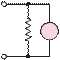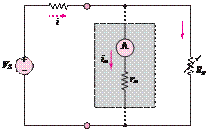### Create an Account

Already have account?

### Forgot Your Password ?

Home / Questions / A circuit that measures the internal resistance of a practical ammeter is shown in Figure...

# A circuit that measures the internal resistance of a practical ammeter is shown in Figure P274 where RS 50000 Q VS 12 V and Rp is a variable resistor that can be adjusted at will a Assume

A circuit that measures the internal resistance of a practical ammeter is shown in Figure P2.74, where R= 50,000 QV= 12 V, and Ris a variable resistor that can be adjusted at will.

a. Assume that r« 50,000 Q. Estimate the current i.

b. If the meter displays a current of 150 µA when

R= 15 Q, find the internal resistance of the

I= 120 mA. Find the voltage across R4 with and without the voltmeter in the circuit for the following values:

a. R4 = 100 Q

b. R4 = 1 kQ

c. R4 = 10 kQ

d.R4 = 100 kQ

meter r.

I120 kÙ VRS 4

Figure P2.74Figure P2.77

View less »

Jun 18 2020 View more View Less

#### Answer (Solved)Subscribe To Get Solution